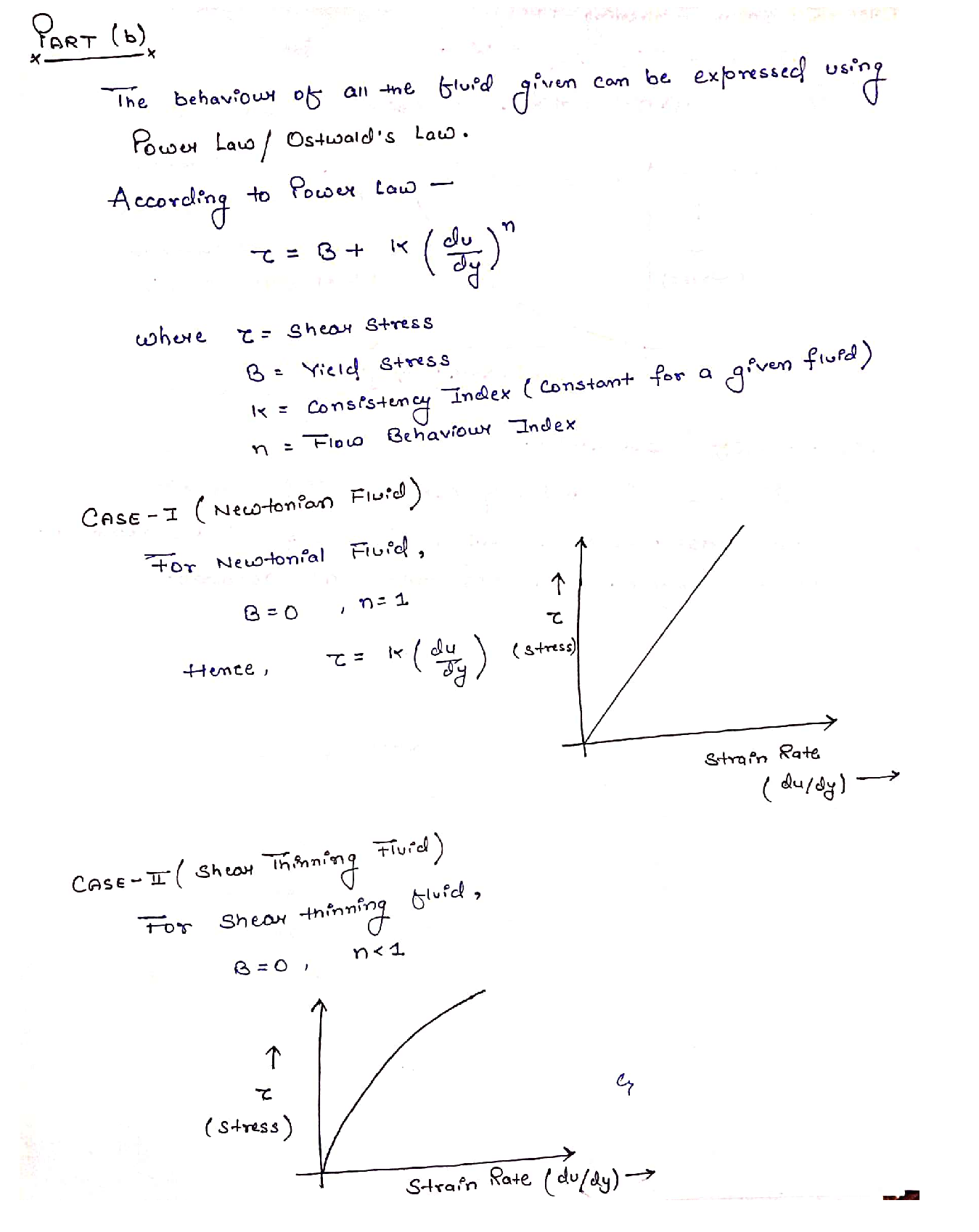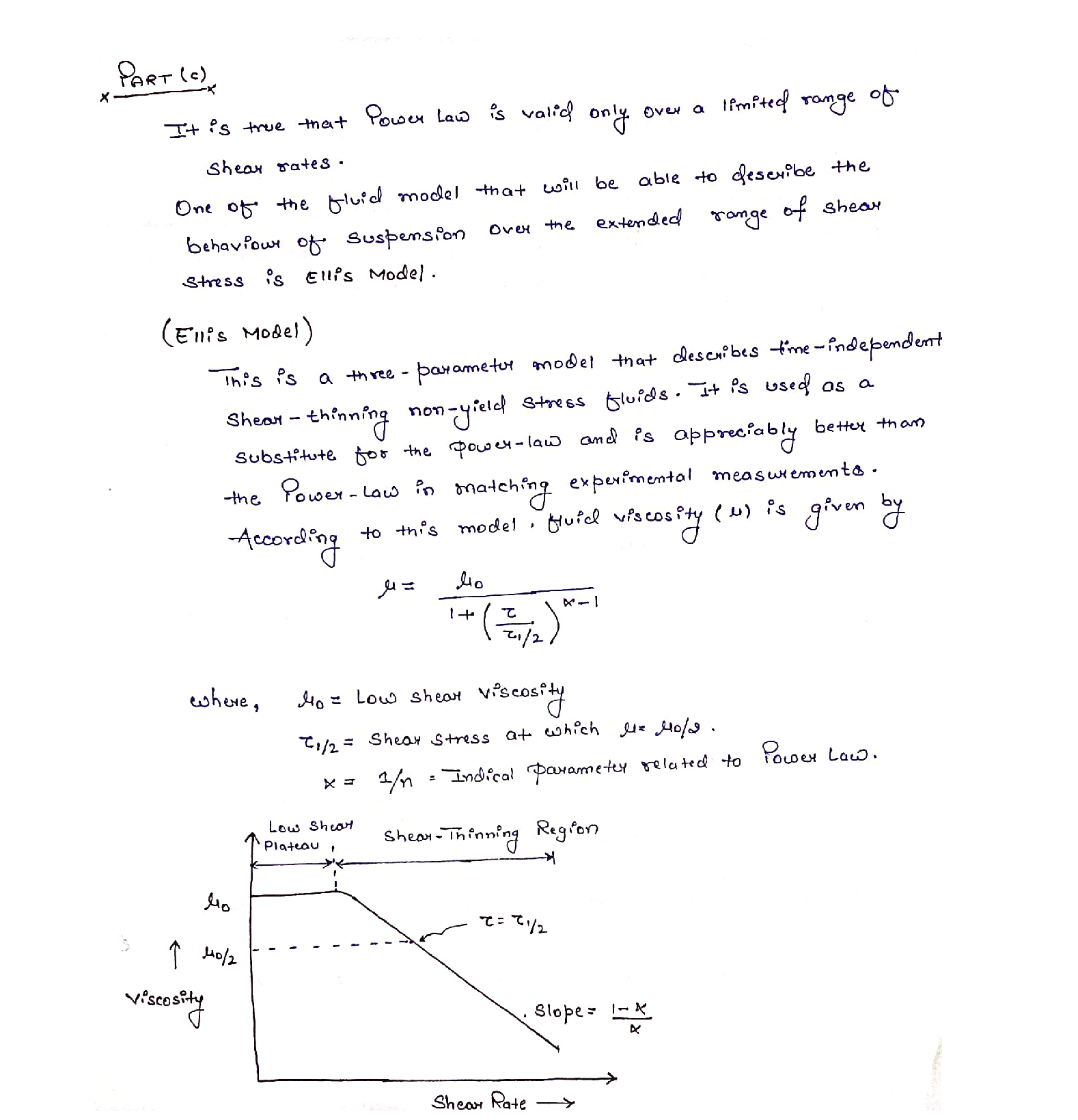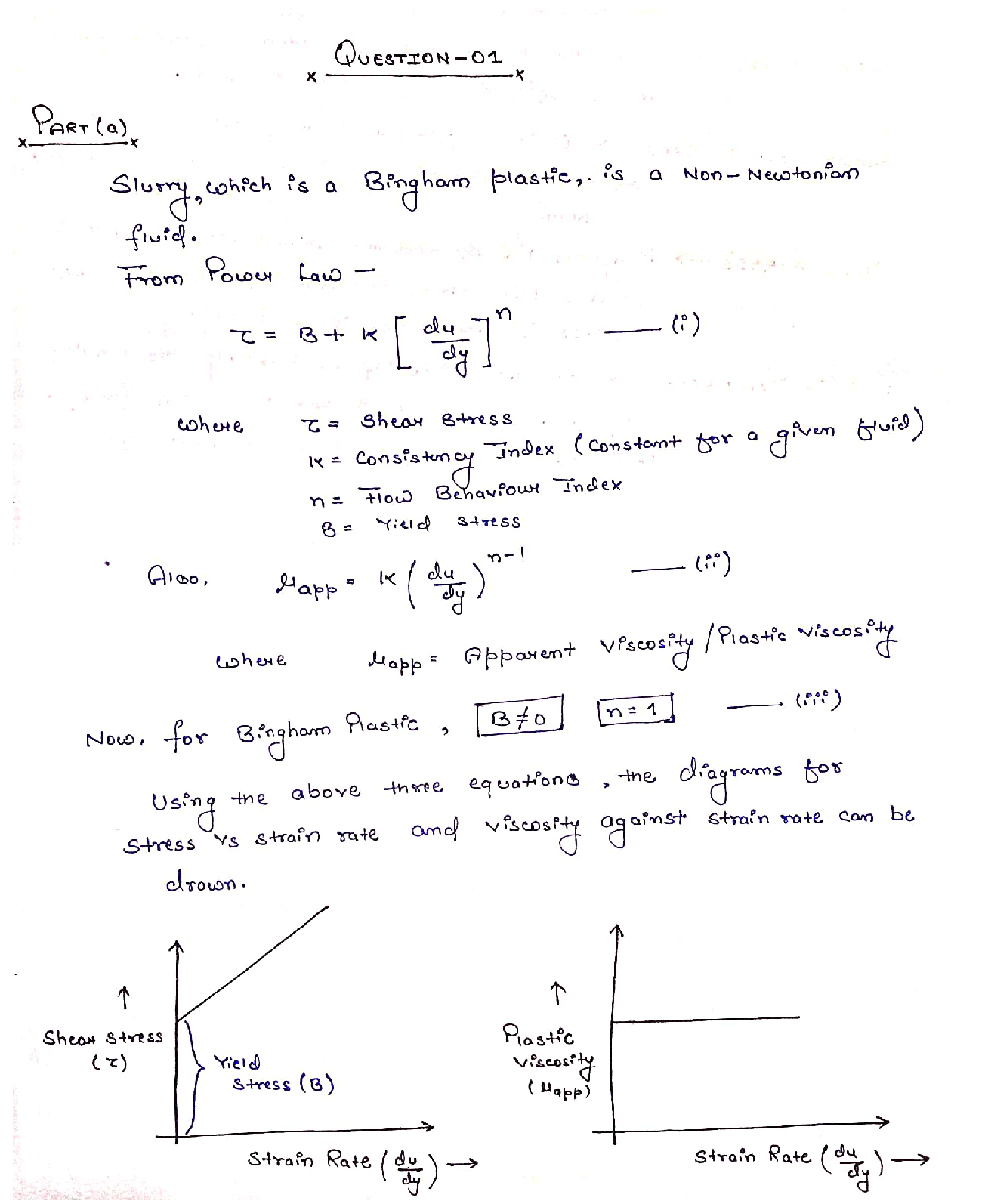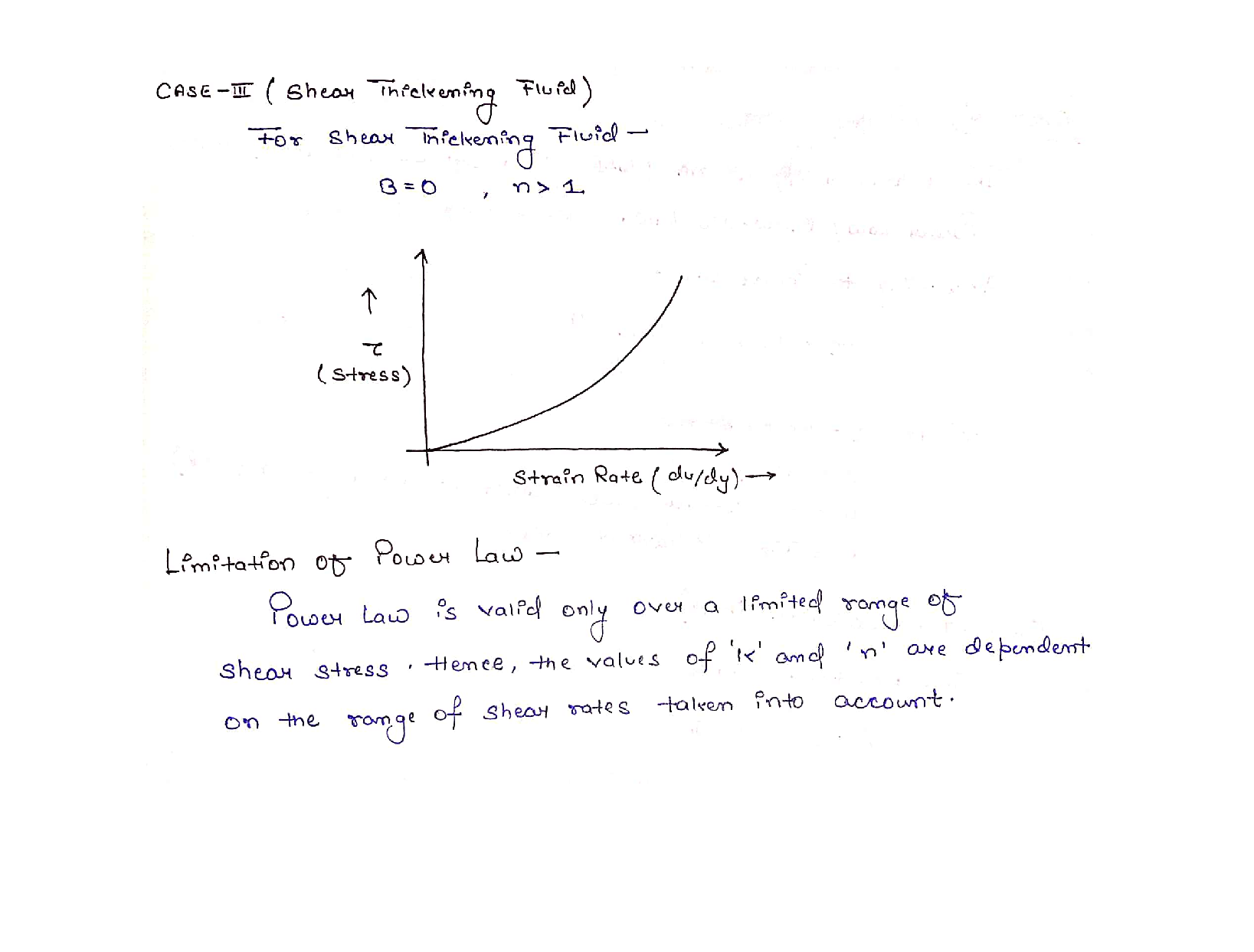Question

Fluid Mechanics

The flow behaviour of a slurry is found to be adequately described by the Bingham Plastic model. Using diagrams of stress vs strain rate and viscosity against strain rate, describe the flow properties of the slurry. Ensure that you include a mathematical expression of the flow behaviour and fully define the terms shear stress, shear strain rate, yield stress and plastic viscosity in your answer.1..7

Suggest a single fluid model that is able to describe the flow characteristics of fluids displaying all of the following fluid behaviours:

Newtonian

Shear thinning

Shear thickening.

Discuss how the model is applied to each type of fluid behaviour and any limitations of using your suggested model. Include a plot of stress vs strain rate for each of the fluid behaviours.

=)A suspension is found to exhibit shear thinning behaviour described by the power law model over the range of experimentally accessible strain rates. It is suggested that the range of shear rates can be extended to include higher strain rates using a complementary experimental technique. Suggest a fluid model that will be able to describe the behaviour of the suspension over the extended range of shear rates. Include the mathematical expression of your chosen model and a plot of apparent viscosity vs strain rate.Verified### Question 45484Fluid Mechanics

Water in the Belle Fourche Reservoir in Western South Dakotais transported to the water storage reservoir for the town of-Buffalo through a series of three pipes, all with friction factors of 0.02. The first pipe is 500 meters long with a diameter of 0.4meters; the second pipe is 600 meters long with a diameter of0.3 meters; and the third pipe is 500 meters long with a diameter of 0.2 meters. The water surface elevation of the Belle FourcheReservoir is 30 meters higher than the town's storage reservoir.Since the current piping is almost 60 years old, the town of Buffalo has decided to replace the piping system with a single pipe of constant diameter. You have been chosen to calculate the equivalent diameter for the single pipe design and determine the flow rate that will occur. Go to work.

### Question 44899Fluid Mechanics

Transmission lines

### Question 44896Fluid Mechanics

A boat has a volume of 40.0 m³ and a mass of 2.00 tons. What load will push 75.0% of its volume into water? Each Metric ton is 1000 kg.

### Question 44895Fluid Mechanics

A tank has a height of 800 mm and a length of 500 mm. The tank contains a liquid of RD 0.9.Determine:
a. The magnitude of the force on the side of the tank per meter width and its position relative to the base of the tank
b. The force on the base per meter width of tank.

### Question 44894Fluid Mechanics

A closed tank contains three different fluids with a vapor space above the oil, as shown in the figure below. Pressure gauge 1 reads the gauge pressure of the vapor space (P1) and pressure gauge 2 reads the pressure at the bottom of the tank (P2). When P1 = 25 kPa, determine:
a. Height of glycerine (h) in the tank if P2 = 95.66 kPa
. P2 reading if glycerine is replaced with water

### Question 44805Fluid Mechanics

Water flows into cylindrical tank through inlet A and D at rate of 20 ft/s and 5 ft/s respectively. If waterleaves through outlet B and C at rate of 3 ft/s and 10 ft/s respectively.
(A) Derive the continuity equation
\text { Know that } D_{A}=2.5 \text { in, } D_{D}=2 \text { in } D_{B}=3 \text { in and } D_{C}=3.5 \text { in. }
(B) Calculate the change of high h?

### Question 44804Fluid Mechanics

An object which has cubic shape is been floating on the water surface see the diagram below. If the object density is less than water, density find the ratio X/L?

### Question 44803Fluid Mechanics

A force of 15.83 kN is exerted on a piston B (diameter = 250 mm) which fits into a cylinder see the diagram below. How much force is need on piston C to start displacing piston B and Mercury on piston B?

### Question 44802Fluid Mechanics

Given the diagram below if the pressure gauge reads 6.1 psig find Y and X in ft. Given that
\mathrm{SG}_{\mathrm{Aloohal}}=0.9, \mathrm{SG}_{\mathrm{Hg}}=13.6, \text { Alcohol vapor } P=1.7 \mathrm{psig}, Z=4 \mathrm{ft} \text { and } \mathrm{Y}_{\mathrm{H} 2 \mathrm{o}}=62.4 \mathrm{\textrm {lb }} / \mathrm{ft}^{3}

### Question 44801Fluid Mechanics

Given the diagram below, calculate the pressure at A, B, C.
\text { Given that } \mathrm{Y} \text { н2о }=9.79 \mathrm{kN} / \mathrm{m}

### Submit query

Getting answers to your urgent problems is simple. Submit your query in the given box and get answers Instantly.

### Submit a new QuerySuccess

Assignment is successfully created